## Relatively Prime

Two integers are relatively prime if they share no common factors (divisors) except 1. Using the notation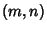to denote the Greatest Common Divisor, two integersandare relatively prime if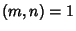. Relatively prime integers are sometimes also called Strangers or Coprime and are denoted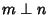.

The probability that two Integers picked at random are relatively prime is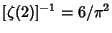, where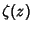is the Riemann Zeta Function. This result is related to the fact that the Greatest Common Divisor ofand,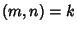, can be interpreted as the number of Lattice Points in the Plane which lie on the straight Line connecting the Vectors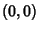and(excludingitself). In fact,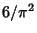is the fractional number of Lattice Points Visible from the Origin (Castellanos 1988, pp. 155-156).

Given three Integers chosen at random, the probability that no common factor will divide them all is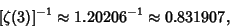(1)

where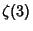is Apéry's Constant. This generalizes to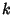random integers (Schoenfeld 1976).

References

Castellanos, D. The Ubiquitous Pi.'' Math. Mag. 61, 67-98, 1988.

Guy, R. K. Unsolved Problems in Number Theory, 2nd ed. New York: Springer-Verlag, pp. 3-4, 1994.

Schoenfeld, L. Sharper Bounds for the Chebyshev Functions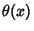and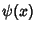, II.'' Math. Comput. 30, 337-360, 1976.